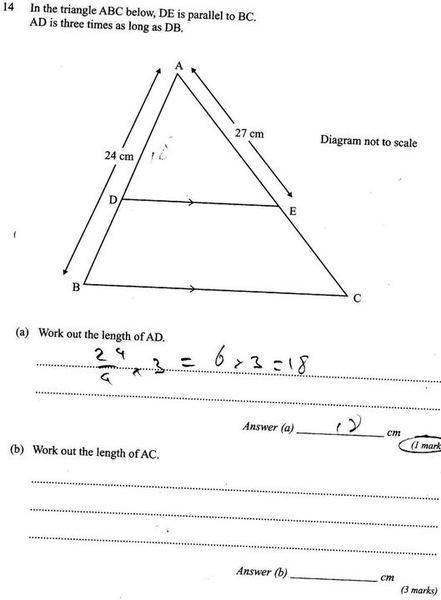# How are these similar

thomas49thI solved both questions, but how do I know that the triangle were similar, Im guessing it's somthing to do with the parallel lines...

ASA
SSS
SAS
RHS

^ I thought these were the ways to spot similar triangles...

## Answers and Replies

neutrino
It's been a long time since I came across those abbreviations, and I don't remember most of it. But you can see that AB and AC are transversals intersecting the parallel lines. Thus angles ADE = ABC and and angles AED = ACB. And, of course, angle A is common to both.

Edit: I now recollect... Angle-Side-Angle, Side-Side-Side... :)

thomas49th
and DAE = BAC and AEC = BAC

so is it AAA -- is there such a similar/congrucy thingy ma jig?

drpizza
ASA, SAS, SSS, etc, aren't to find that triangles are *similar* - they are for showing the triangles are *congruent* (same size)

To know the triangles are similar, you only need to know that two of the corresponding pairs of angles are congruent (because it follows that since the sum of the angles in a triangle is 180 degrees, the 3rd pair of corresponding angles would also have to be congruent.) Thus, AA is all that's needed for *similar* triangles.
Obviously, angle A is congruent to angle A (reflexive property)
You can do either or both of the other pair of corresponding angles just as you mentioned - it has to do with the parallel lines. "When a pair of parallel lines are cut by a transversal, the corresonding angles are congruent."